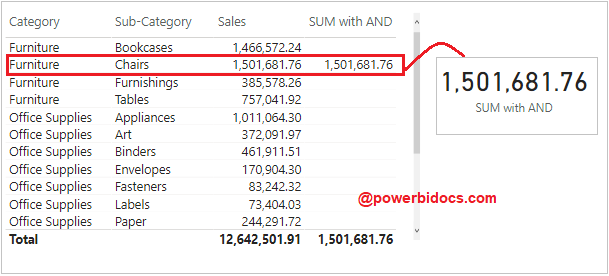Home » DAX » AND DAX Function

# AND DAX FunctionChecks whether both arguments are TRUE, and returns TRUE if both arguments are TRUE. Otherwise returns false. Its comes under Logical DAX functions category.

#### Syntax:

`AND(logical1, logical2)`

#### Parameters

logical1, logical2 – The logical values you want to test.

#### Let’s understand with an example:

See the below sample data, here we have Category, sub category and Sales data.Sample Data

Suppose you are interested to see the sales only where Category = “Furniture” and Sub Category =”Chairs”.

In order to achieve this you can use AND DAX function, it will check both conditions are true or not, if both conditions are TRUE then returns TRUE otherwise returns false.

Now create one measure for AND Dax function-

SUM with AND =
CALCULATE(
SUM(‘Global-Superstore'[Sales]),
FILTER (‘Global-Superstore’,
AND (
‘Global-Superstore'[Category] = “Furniture”,
‘Global-Superstore'[Sub-Category]=“Chairs”
)
)
)

Now drag measure to Table and in Card visual, it will return the sum only for whether both conditions are true.DAX- SUM with AND

### Another way to write AND function-

Instead of AND you can use && sign, let’s have a look in below DAX code.

```SUM with AND = CALCULATE(
SUM('Global-Superstore'[Sales]),
FILTER (
'Global-Superstore',
'Global-Superstore'[Category] = "Furniture" &&
'Global-Superstore'[Sub-Category]="Chairs"
) )```

Refer more DAX functions: DAX functions

Hope you enjoyed the post. Your valuable feedback, question, or comments about this post are always welcome or you can leave us message on our Contact form , we will revert to you asap.